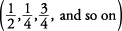## Mixed Numbers

When a term contains both a whole number (3, 8, 25, and so on) and a fraction, the term is called a mixed number. For instance,andare both mixed numbers.

#### Changing improper fractions

To change an improper fraction to a mixed number, you divide the denominator into the numerator.

##### Example 1

Changeto a mixed number.#### Changing mixed numbers

To change a mixed number to an improper fraction, you multiply the denominator times the whole number, add in the numerator, and put the total over the original denominator.

##### Example 2

Changeto an improper fraction.